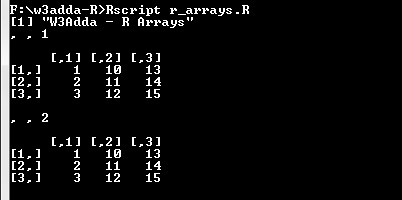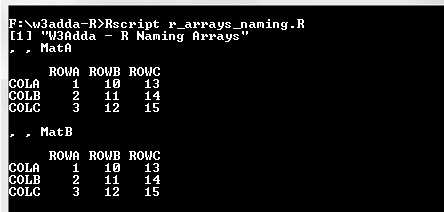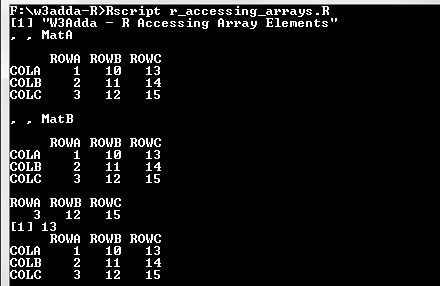# R Arrays

In this tutorial you will learn about the R Arrays and its application with practical example.

## R Arrays

Array is a data structure that enable you to store multi dimensional(more than 2 dimensions) data of same type. In R, an array of (2, 3, 2) dimension contains 2 matrix of 2×3.

## Creating Arrays

In R, an array can be created using the array() function as following –

Syntax:-

Above is the general syntax of array() function, here

data:- It is a vector input used to create array.
dim:- It is used to represent array dimensions.

Example:-

Output:-## Naming Columns and Rows

The dimnames parameter in array() function is used to give names to the rows, columns along with matrices.

Example:-

Output:-## Accessing Array Elements

Array elements can be accessed by using the row and column index of the element as following –

Syntax:-

arr:- Array name
row:- row index of the element, if not provided specified row elements will be fetched for all columns.
col:- column index of the element, if not provided specified column elements will be fetched for all rows.
matrix:- matrix index.

Example:-

When we run the above R script, we see the following output –

Output:-In this tutorial we have learn about the R Arrays and its application with practical example. I hope you will like this tutorial.